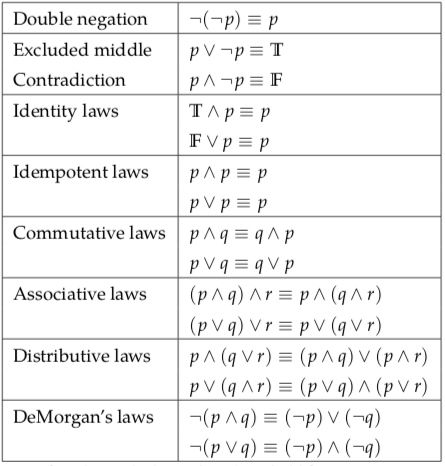# 1.2: Boolean Algebra

$$\newcommand{\vecs}{\overset { \rightharpoonup} {\mathbf{#1}} }$$ $$\newcommand{\vecd}{\overset{-\!-\!\rightharpoonup}{\vphantom{a}\smash {#1}}}$$$$\newcommand{\id}{\mathrm{id}}$$ $$\newcommand{\Span}{\mathrm{span}}$$ $$\newcommand{\kernel}{\mathrm{null}\,}$$ $$\newcommand{\range}{\mathrm{range}\,}$$ $$\newcommand{\RealPart}{\mathrm{Re}}$$ $$\newcommand{\ImaginaryPart}{\mathrm{Im}}$$ $$\newcommand{\Argument}{\mathrm{Arg}}$$ $$\newcommand{\norm}{\| #1 \|}$$ $$\newcommand{\inner}{\langle #1, #2 \rangle}$$ $$\newcommand{\Span}{\mathrm{span}}$$ $$\newcommand{\id}{\mathrm{id}}$$ $$\newcommand{\Span}{\mathrm{span}}$$ $$\newcommand{\kernel}{\mathrm{null}\,}$$ $$\newcommand{\range}{\mathrm{range}\,}$$ $$\newcommand{\RealPart}{\mathrm{Re}}$$ $$\newcommand{\ImaginaryPart}{\mathrm{Im}}$$ $$\newcommand{\Argument}{\mathrm{Arg}}$$ $$\newcommand{\norm}{\| #1 \|}$$ $$\newcommand{\inner}{\langle #1, #2 \rangle}$$ $$\newcommand{\Span}{\mathrm{span}}$$$$\newcommand{\AA}{\unicode[.8,0]{x212B}}$$

So far we have discussed how to write and interpret propositions. This section deals with manipulating them. For this, we need algebra. Ordinary algebra, of the sort taught in high school, is about manipulating numbers, variables that represent numbers, and operators such as + and × that apply to numbers. Now, we need an algebra that applies to logical values, propositional variables, and logical operators. The first person to think of logic in terms of algebra was the mathematician, George Boole, who introduced the idea in a book that he published in 1854. The algebra of logic is now called Boolean algebra in his honour.

George Boole (1815–1864) was a largely self-taught British mathematician, philosopher and logician, most of whose short career was spent as the first professor of mathematics at Queen’s College, Cork in Ireland. He worked in the fields of differential equations and algebraic logic, and is best known as the author of The Laws of Thought (1854). Among TU Delft students he is best known for the room named after him in the EEMCS building 28.Boolean logic is credited with laying the foundations for the information age: essentially, computer science. Boole maintained that:

“No general method for the solution of questions in the theory of probabilities can be established which does not explicitly recognize, not only the special numerical bases of the science, but also those universal laws of thought which are the basis of all reasoning, and which, whatever they may be as to their essence, are at least mathematical as to their form.”

Source: en.Wikipedia.org/wiki/George_Boole.

The algebra of numbers includes a large number of rules for manipulating expressions. The distributive law, for example, says that x(y + z) = xy + xz, where x, y, and zare variables that stand for any numbers or numerical expressions. This law means that whenever you see something of the form xy + xz in a numerical expression, you can substitute x(y + z) without changing the value of the expression, and vice versa. Note that the equals sign in x(y + z) = xy + xz means “has the same value as, no matter what numerical values x, y, and z have”.

In Boolean algebra, we work with logical values instead of numerical values. There are only two logical values, true and false. We will write these values as T and F or 1 and 0. The symbols T and F play a similar role in Boolean algebra to the role that constant numbers such as 1 and 3.14159 play in ordinary algebra. Instead of the equals sign, Boolean algebra uses logical equivalence, ≡, which has essentially the same meaning.Figure 2.2: Laws of Boolean Algebra. These laws hold for any propositions p, q, and r.

For example, for propositions p, q, and r, the ≡ operator in p∧(qr) ≡ (pq)∧rmeans “has the same value as, no matter what logical values p, q, and r have”.

This page titled 1.2: Boolean Algebra is shared under a CC BY-NC-SA 4.0 license and was authored, remixed, and/or curated by Stefan Hugtenburg & Neil Yorke-Smith (TU Delft Open) via source content that was edited to the style and standards of the LibreTexts platform; a detailed edit history is available upon request.